International
Tables for
Crystallography
Volume B
Reciprocal space
Edited by U. Shmueli

International Tables for Crystallography (2010). Vol. B, ch. 5.3, pp. 654-655   | 1 | 2 |

## Section 5.3.2. Comparison between X-rays and neutrons with spin neglected

M. Schlenkera* and J.-P. Guigayb

aLaboratoire Louis Néel du CNRS, BP 166, F-38042 Grenoble Cedex 9, France, and  bEuropean Synchrotron Radiation Facility, BP 220, F-38043 Grenoble, France
Correspondence e-mail:  schlenk@grenoble.cnrs.fr

### 5.3.2. Comparison between X-rays and neutrons with spin neglected

| top | pdf |

#### 5.3.2.1. The neutron and its interactions

| top | pdf |

An excellent introductory presentation of the production, properties and scattering properties of neutrons is available (Scherm & Fåk, 1993, and other papers in the same book). A stimulating review on neutron optics, including diffraction by perfect crystals, has been written by Klein & Werner (1983). X-rays and neutrons are compared in terms of the basic quantities in Table 4.1.3.1of IT C (2004), where Chapter 4.4is devoted to neutron techniques.

The neutron is a massive particle for which the values relevant to diffraction are: no electric charge, rest mass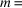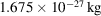, angular momentum eigenvalues along a given direction(spin) and a magnetic moment of −1.913 nuclear magneton, meaning that its component along a quantization direction z can take eigenvalues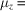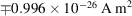. The de Broglie wavelength iswhere h is Planck's constant () and p is the linear momentum;in the nonrelativistic approximation, which always applies in the context of this chapter, v being the neutron's velocity. The neutron's wavelength, λ, and kinetic energy,, are thus related by, or, in practical units,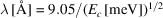. Thus, to be of interest for diffraction by materials, neutrons should have kinetic energies in the rangetomeV. In terms of the velocity,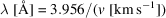.

Neutron beams are produced by nuclear reactors or by spallation sources, usually pulsed. In either case they initially have an energy in the MeV range, and have to lose most of it before they can be used. The moderation process involves inelastic inter­actions with materials. It results in statistical distributions of energy, hence of velocity, close to the Maxwell distribution characteristic of the temperature T of the moderator. Frequently used moderators are liquid deuterium (D2, i.e. 2H2) at 25 K, heavy water (D2O) at room temperature and graphite allowed to heat up to 2400 K; the corresponding neutron distributions are termed cold, thermal and hot, respectively.

The interaction of a neutron with an atom is usually described in terms of scattering lengths or of scattering cross sections. The main contribution corresponding to the nuclear interaction is related to the strong force. The interaction with the magnetic field created by atoms with electronic magnetic moments is comparable in magnitude to the nuclear term.

#### 5.3.2.2. Scattering lengths and refractive index

| top | pdf |

The elastic scattering amplitude for scattering vector s,, is defined by the wave scattered by an object placed at the origin when the incident plane wave is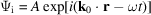, written as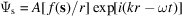with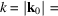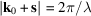. In the case of the strong-force interaction with nuclei, the latter can be considered as point scatterers because the interaction range is very small, hence the scattering amplitude is isotropic (independent of the direction of s). It is also independent of λ except in the vicinity of resonances. It is conventionally written as −b so that most values of b, called the scattering length, are positive. A table of experimentally measured values of the scattering lengths b is given in IT C for the elements in their natural form as well as for many individual isotopes. It is apparent that the typical order of magnitude is the fm (femtometre, i.e.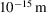, or fermi), that there is no systematic variation with atomic number and that different isotopes have very different scattering lengths, including different signs. The first remark implies that scattering amplitudes of X-rays and of neutrons have comparable magnitudes, because the characteristic length for X-ray scattering (the scattering amplitude for forward scattering by one free electron) isfm, the classical electron radius. The second and third points explain the importance of neutrons in structural crystallography, in diffuse scattering and in small-angle scattering. Scattering of neutrons by condensed matter implies the use of the bound scattering lengths, as tabulated in IT C. The free' scattering length, used in some presentations, is obtained by multiplying the bound scattering lengths by, where A is the mass of the nucleus in atomic units.

A description in terms of an interaction potential is possible using the Fermi pseudo-potential, which in the case of the nuclear interaction with a nucleus atcan be written as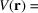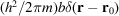, where δ denotes the three-dimensional Dirac distribution.

Refraction of neutrons at an interface can be conveniently described by assigning a refractive index to the material, such that the wavenumber in the material, k, is related to that in a vacuum,, by. Herewhere the sum is over the nuclei contained in volume V. With typical values, n is very close to 1 andis typically of the order of. This small value, in the same range as for X-rays, gives a feeling for the order of magnitude of key quantities of the dynamical theory, in particular the Darwin widthas discussed in Chapter 5.1. It also makes total external reflection possible on materials for which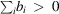: this is the basis for the neutron guide tubes now installed in most research reactors, as well as for reflectometry.

The notations prevailing in X-ray and in neutron crystallography are slightly different, and the correspondence is very simple: X-ray atomic scattering factors and structure factors are numbers. When multiplied by R, the classical electron radius, they become entirely equivalent to the corresponding quantities in neutron usage, which are lengths. It should be noted that the presence of different isotopes and the effect of nuclear spin (disordered except under very special conditions) give rise to incoherent elastic neutron scattering, which has no equivalent in the X-ray case. The scattering length corresponding to R times the atomic scattering factor for X-rays is therefore the coherent scattering length,, obtained by averaging the scattering length over the nuclear spin state and isotope distribution.

#### 5.3.2.3. Absorption

| top | pdf |

Neutron absorption is related to a nuclear reaction in which the neutron combines with the absorbing nucleus to form a compound nucleus, usually in a metastable state which then decays. The scattering length describing this resonance scattering process depends on the neutron energy and contains an imaginary part associated with absorption in complete analogy with the imaginary part of the dispersion correction for the X-ray atomic scattering factors. The energies of the resonances are usually far above those of interest for crystallography, and the linear absorption coefficient varies approximately asor λ. It is important to note that, except for a very few cases (notably 3He, 6Li, 10B, In, Cd, Gd), the absorption of neutrons is very small compared with that of X-rays, and even more so compared with that of electrons, and can be neglected to a first approximation.

#### 5.3.2.4. Differences between neutron and X-ray scattering

| top | pdf |

There are major differences in the experimental aspects of neutron and X-ray scattering. Neutrons are only available in large facilities, where allocation of beam time to users is made on the basis of applications, and where admittance is restricted because of the hazards which nuclear technology can present in the hands of ill-intentioned users. Because of the radiation shielding necessary, as well as the large size of neutron detectors, neutron-scattering instrumentation is much bulkier than that for X-rays. Neutron beams are in some aspects similar to synchrotron radiation, in particular because in both cases the beams are initially white' and for most applications have to be monochromated. There is, however, a huge difference in the order of magnitudes of the intensities. Neutron beams are weak in comparison with laboratory X-ray sources, and weaker by many orders of magnitude than synchrotron radiation. Also, the beam sources are large in the case of neutrons, since they are essentially the moderators, whereas the source is very small in the case of synchrotron radiation, and this difference again increases the ratio of the brilliances in favour of X-rays. This encourages the use of large specimens in all neutron-scattering work, and makes the extinction problem more important than for X-rays. Furthermore, many experiments that are quick using X-rays become very slow, and give rise to impaired resolution, in the neutron case.

There are also at least two additional aspects of neutron scattering in comparison with X-ray scattering, apart from the effect of the magnetic moment associated with the intrinsic (spin) angular momentum of the neutron. On the one hand, the small velocity of neutrons, compared with the velocity of light, makes time-of-flight measurements possible, both in standard neutron diffraction and in investigations of perfect crystals. Because this velocity is of the same order of magnitude as that of ultrasound, the effect of ultrasonic excitation on neutron diffraction is slightly different from that in the X-ray case. On the other hand, the fact that neutrons have mass and a magnetic moment implies that they can be affected by external fields, such as gravity and magnetic fields, both during their propagation in air or in a vacuum and while being diffracted within crystals (Werner, 1980) (see Section 5.3.5). Experiments completely different from the X-ray case can thus be performed with perfect crystals and with neutron interferometers (see Sections 5.3.6and 5.3.7.3).

#### 5.3.2.5. Translating X-ray dynamical theory into the neutron case

| top | pdf |

As shown in Chapter 5.1, the basic equations of dynamical theory, viz Maxwell's equations for the X-ray case and the time-independent Schrödinger equation in the neutron case, have exactly the same form when the effect of the neutron spin can be neglected, i.e. in situations that do not involve magnetism and when no externally applied potential is taken into account. The translation scheme for the scattering factors and structure factors is described above. The one formal difference is that the wavefunction is scalar in the neutron case, hence there is no equivalent to the parallel and perpendicular polarizations of the X-ray situation: C in equation (5.1.2.20) of Chapter 5.1 should therefore be set to 1.

The physics of neutron diffraction by perfect crystals is therefore expected to be very similar to that of X-ray diffraction, with the existence of wavefields, Pendellösung effects, anomalous transmission, intrinsic rocking-curve shapes and reflectivity versus thickness behaviour in direct correspondence. All experimental tests of these predictions confirm this view (Section 5.3.6).

Basic discussions of dynamical neutron scattering are given by Stassis & Oberteuffer (1974), Sears (1978), Rauch & Petrascheck (1978)and Squires (1978).

### References

International Tables for Crystallography (2004). Vol. C. Mathematical, Physical and Chemical Tables, edited by E. Prince. Dordrecht: Kluwer Academic Publishers.
Klein, A. G. & Werner, S. A. (1983). Neutron optics. Rep. Prog. Phys. 46, 259–335.
Rauch, H. & Petrascheck, D. (1978). Dynamical neutron diffraction and its application. In Neutron Diffraction, edited by H. Dachs, Topics in Current Physics, Vol. 6 pp. 305–351. Berlin: Springer.
Scherm, R. & Fåk, B. (1993). Neutrons. In Neutron and Synchrotron Radiation for Condensed Matter Studies (HERCULES Course), Vol. 1, edited by J. Baruchel, J. L. Hodeau, M. S. Lehmann, J. R. Regnard & C. Schlenker, pp. 113–143. Les Ulis: Les Editions de Physique and Heidelberg: Springer-Verlag.
Sears, V. F. (1978). Dynamical theory of neutron diffraction. Can. J. Phys. 56, 1261–1288.
Squires, G. L. (1978). Introduction to the Theory of Thermal Neutron Scattering. Cambridge University Press.
Stassis, C. & Oberteuffer, J. A. (1974). Neutron diffraction by perfect crystals. Phys. Rev. B, 10, 5192–5202.
Werner, S. A. (1980). Gravitational and magnetic field effects on the dynamical diffraction of neutrons. Phys. Rev. B, 21, 1774–1789.